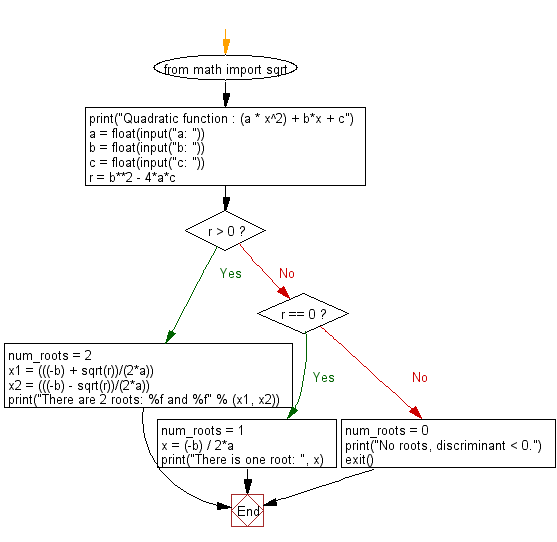﻿ Python Math: Find the roots of a quadratic function - w3resource# Python Math: Find the roots of a quadratic function

## Python Math: Exercise-30 with Solution

Write a Python program to find the roots of a quadratic function.

Sample Solution:-

Python Code:

``````from math import sqrt

print("Quadratic function : (a * x^2) + b*x + c")
a = float(input("a: "))
b = float(input("b: "))
c = float(input("c: "))

r = b**2 - 4*a*c

if r > 0:
num_roots = 2
x1 = (((-b) + sqrt(r))/(2*a))
x2 = (((-b) - sqrt(r))/(2*a))
print("There are 2 roots: %f and %f" % (x1, x2))
elif r == 0:
num_roots = 1
x = (-b) / 2*a
print("There is one root: ", x)
else:
num_roots = 0
print("No roots, discriminant < 0.")
exit()
```
```

Sample Output:

```Quadratic function : (a * x^2) + b*x + c
a: 5
b: 20
c: 10
There are 2 roots: -0.585786 and -3.414214
```

Flowchart:## Visualize Python code execution:

The following tool visualize what the computer is doing step-by-step as it executes the said program:

Python Code Editor:

Have another way to solve this solution? Contribute your code (and comments) through Disqus.

What is the difficulty level of this exercise?

Test your Programming skills with w3resource's quiz.

﻿

```>>> students = [{'name': 'John', 'score': 98}, {'name': 'Mike', 'score': 94}, {'name': 'Jennifer', 'score': 99}]